# The Transportation Problem in AMPL

## AMPL Formulation

The formulation of the transportation problem is AMPL is a straightforward translation of the mathematical programme for the transportation problem.

The sets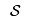and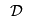are declared as SUPPLY_NODES and DEMAND_NODES respectively:

set SUPPLY_NODES;
set DEMAND_NODES;


The supply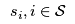and demand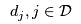are declared as integer parameters:

param Supply {SUPPLY_NODES} >= 0, integer;
param Demand {DEMAND_NODES} >= 0, integer;


The cost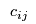is declared over the SUPPLY_NODES and DEMAND_NODES:

param Cost {SUPPLY_NODES, DEMAND_NODES};


Now, the mathematical programme follows directly:

var Flow {SUPPLY_NODES, DEMAND_NODES} >= 0, integer;

minimize TotalCost:
sum {i in SUPPLY_NODES, j in DEMAND_NODES} Cost[i, j] * Flow[i, j];

subject to UseSupply {i in SUPPLY_NODES}:
sum {j in DEMAND_NODES} Flow[i, j] = Supply[i];

subject to MeetDemand {j in DEMAND_NODES}:
sum {i in SUPPLY_NODES} Flow[i, j] = Demand[j];

Note that we assume the transportation is balanced.

In the main discussion of transportation problems, we saw that adding bounds to the flow variables allowed us to easily either bound the transportation of good from a supply node to a demand node or remove an arc from the problem altogether.

We can add bounds to our AMPL formulation by declaring 2 new parameters with defaults:

param Lower {SUPPLY_NODES, DEMAND_NODES} integer default 0;
param Upper {SUPPLY_NODES, DEMAND_NODES} integer default Infinity;

and adding them to the Flow variable declaration:
var Flow {i in SUPPLY_NODES, j in DEMAND_NODES}
>= Lower[i, j], <= Upper[i, j], integer;


## Balancing Transportation Problems

Balanced transportation models are preferred as there is no confusion about the relational operators for the supply and demand constraints. We can use script file to balance any transportation problem automatically:

reset;

model transportation.mod;

param costFromDummy {DEMAND_NODES} default 0;
param costToDummy   {SUPPLY_NODES} default 0;

param difference;

# Add the problem date file here
# e.g., data brewery.dat;

let difference := (sum {s in SUPPLY_NODES} Supply[s])
- (sum {d in DEMAND_NODES} Demand[d]);

if difference > 0 then
{
let DEMAND_NODES := DEMAND_NODES union {'Dummy'};
let Demand['Dummy'] := difference;
let {s in SUPPLY_NODES} Cost[s, 'Dummy'] := costToDummy[s];
}
else if difference < 0 then
{
let SUPPLY_NODES := SUPPLY_NODES union {'Dummy'};
let Supply['Dummy'] := - difference;
let {d in DEMAND_NODES} Cost['Dummy', d] := costFromDummy[d];
}; # else the problem is balanced

# Make sure the problem is balanced
check : sum {s in SUPPLY_NODES} Supply[s] = sum {d in DEMAND_NODES} Demand[d];

option solver cplex;

solve;

display Flow;

Note the check statement to ensure that the balancing has been done properly before solving. Also, note that costToDummy and costFromDummy allow for the definition of costs on any flow from/to a dummy node in the data file.

Both the AMPL model file transportation.mod and script file transportation.run are attached.

-- MichaelOSullivan - 02 Apr 2008

Topic attachments
I Attachment History Action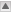Size Date Who Comment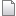mod transportation.mod r1 manage 1.2 K 2008-04-02 - 10:41 MichaelOSullivanrun transportation.run r1 manage 0.9 K 2008-04-02 - 10:42 MichaelOSullivan

This topic: OpsRes > WebHome > AMPLGuide > TransportationProblemInAMPL
Topic revision: r4 - 2008-04-02 - MichaelOSullivan

Copyright © 2008-2021 by the contributing authors. All material on this collaboration platform is the property of the contributing authors.
Ideas, requests, problems regarding TWiki? Send feedback